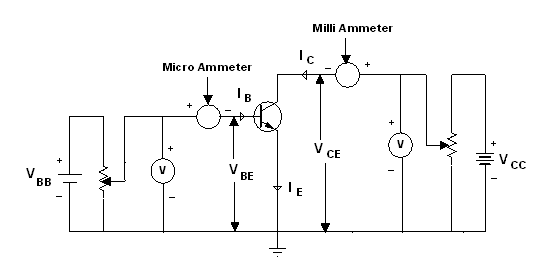# Common Emitter (CE) Configuration of a npn Transistor

Common emitter characteristics of a transistor relate voltage and current when emitter is common to both input and output circuits. VBB is a variable dc supply of 0-3 V and VCC is a variable dc supply of 0-15 V. R1 and R2 are potentiometers and R is a variable resistor. It is used to control base to emitter voltage, VBE.### Input Characteristics

In CE configuration, the input characteristics show the variation of IB with VBE when VCE is held constant. To draw this characteristic, VCE is kept at a suitable value with the help of R and R1. Then VBE is changed in steps and corresponding values of IB are measured with the help of micro ammeter, connected to base.

For a given value of VCE, the curve is as obtained for forward biased p-n junction diode. For VBE < 0.5 V, there is no measurable base current (IB = 0). However, IB rises steeply for VBE > 0.6 V.

From the reciprocal of the slope of input characteristic, you get input resistance of the transistor defined as the ratio of small change in base - emitter voltage to the small change produced in the base current at constant collector - emitter voltage.

Rie = ∆VBE/∆IB

Usually, the value of Rie is in the range 20-100 Ω. Since the curve is not linear, the value of input resistance varies with the point of measurement. As VCE increases, the curve tends to become more vertical and the value of Rie decreases.

### Output Characteristics

The output characteristic curves depict the variation of collector current IC with VCE, when base current IB is kept constant. To draw output characteristics, IB is fixed (say at 10 µA) by adjusting R1 and R.

VCE is then increased from 0 to 10 V in steps of 0.5 V by varying R2 and the corresponding value of IC is noted. Similarly, the output characteristics can be obtained at IB = 40µA, 60µA, 80µA. However, in no case, the maximum base current rating of the transistor should be exceeded.

From the output characteristics, IC changes with increase in VCE for a given value of IB and IC increases with IB for a given VCE.

From these characteristics, you can calculate output admittance (hoe).

hoe = ∆IC/∆VCE